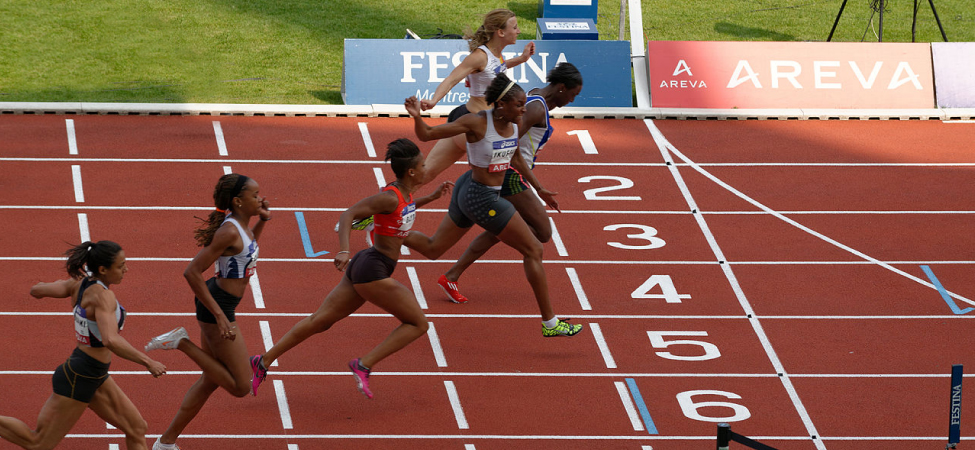Work and Kinetic Energy

# Introduction

A sprinter exerts her maximum power to do as much work on herself as possible in the short time that her foot is in contact with the ground. This adds to her kinetic energy, preventing her from slowing down during the race. Pushing back hard on the track generates a reaction force that propels the sprinter forward to win at the finish. (credit: modification of work by Marie-Lan Nguyen)In this chapter, we discuss some basic physical concepts involved in every physical motion in the universe, going beyond the concepts of force and change in motion, which we discussed in Motion in Two and Three Dimensions and Newton’s Laws of Motion. These concepts are work, kinetic energy, and power. We explain how these quantities are related to one another, which will lead us to a fundamental relationship called the work-energy theorem. In the next chapter, we generalize this idea to the broader principle of conservation of energy.

The application of Newton’s laws usually requires solving differential equations that relate the forces acting on an object to the accelerations they produce. Often, an analytic solution is intractable or impossible, requiring lengthy numerical solutions or simulations to get approximate results. In such situations, more general relations, like the work-energy theorem (or the conservation of energy), can still provide useful answers to many questions and require a more modest amount of mathematical calculation. In particular, you will see how the work-energy theorem is useful in relating the speeds of a particle, at different points along its trajectory, to the forces acting on it, even when the trajectory is otherwise too complicated to deal with. Thus, some aspects of motion can be addressed with fewer equations and without vector decompositions.